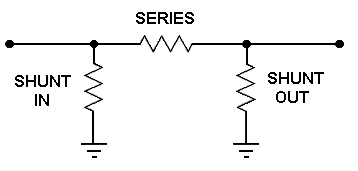# Pi Attenuator Calculator

Calculates the resistor values, attenuation, 'impedance', reflection coefficient, VSWR and return loss of a Pi attenuator. This can be built into a FLEXI-BOX and a transmission line PCB is available which easily adapts for this circuit with one simple trimming operation. There is also a selection of suitable RF connectors available.1) Enter the system impedance and required attenuation then then press "Calculate" below to obtain the Ideal resistor values.

 INPUT DATA System impedance: Ohms Required attenuation: dB
 IDEAL VALUES Ideal series resistor: Ohms Ideal shunt resistor: Ohms

2) Change the calculated resistor values in the amber text boxes below to the nearest Preferred Resistor Values or to those available then press " Calculate" below to get the resulting attenuator parameters.

 SELECTED VALUES Preferred series resistor: Ohms Preferred shunt resistor: Ohms Mismatch Losses: Included
 PARAMETERS Attenuation: dB Input Impedance: Ohms Reflection Coefficient: VSWR: Return Loss: dB

This calculator uses JavaScript and will function in most modern browsers. For more information see About our calculators

The calculator uses simple Ohms law calculations, and more comprehensive equations for Voltage Reflection Coefficient and VSWR which are available at:-

http://www.spectrum-soft.com/news/fall2009/vswr.shtm

This calculator is provided free by Chemandy Electronics in order to promote the FLEXI-BOX